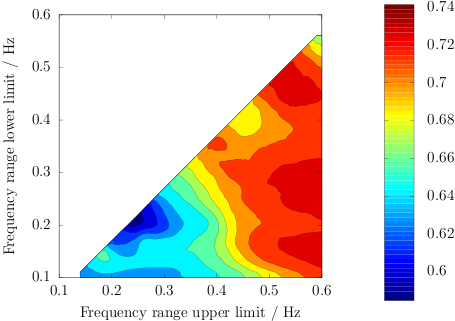### Making gorgeous LaTeX plots with Octave

I previously wrote about using the epslatex terminal in Gnuplot to generate beautiful plots for inclusion in a LaTeX document. The secret is that the epslatex terminal produces a combination of (1) EPS vector graphics and (2) TeX instructions to overlay all the text (axis labels, legends, etc.) in whatever font you are using in the rest of your document. So typically you get that super slick looking Computer Modern Roman (cmr) font.

Now, there are some things that are beyond the ken of Gnuplot. So it was a relief when I learned that GNU Octave can produce similarly formatted EPS + TeX graphics. What's nice about using Octave instead of Gnuplot is that, not only can you take advantage of Octave's more advanced (as I understand it) graphics facilities, but you can also bring to bear all the power of a full mathematical/simulation language for preprocessing your data or whatnot. I still usually use Gnuplot, but I break out Octave for making plots when necessary.

All you have to do is produce your plot in Octave as normal (e.g. plot(...)), and use a command like the following to output in EPS + Tex:

print('my_plot.tex', '-dtex');

As an example, here's some minimal code to produce a heatmap with contours and a legend:

```x_values = [0.10 : 0.005 : 0.60];
y_values = [0.10 : 0.005 : 0.60];
contourf(x_values, y_values, data); % supply your own data...
axis square;
colorbar;
xlim([0.1 0.6]);
ylim([0.1 0.6]);
print('-dtex', 'my_plot.tex');```Octave really saves the day here. To the best of my knowledge it is difficult or impossible to do this using just plain Gnuplot, especially if you are not plotting over a square area.

1.I know this is an old post, but how do you incorporate this into LaTeX? I keep getting errors when I try.

2.I think you want to do \input{path/to/generated/file.tex}. And then because the output is in EPS, you probably have to use regular latex (not pdflatex; use dvipdf if you want to convert to PDF). Let me know if that doesn't work for you.

3.Regarding you last post, Phil - if you then would like to add both png's and this eps file in the same latex file, one is into trouble? Or can these two image types somehow be combined?

4.As far as I know you can't use PNG and EPS in the same file. I think you can however work around this by using Imagemagick or any other conversion tool to convert your PNGs into EPS.

5.Thank you, this was really helpful!

Any ideas on how to scale the figure in a LaTeX doucument, but keep the current fontsize on the axis?
I have tried \scalebox[scale]{\input{plot.tex}} but this will also scale the text.

6.@Ørnulf,

When using gnuplot directly you can do e.g. "set size 1.5, 1.5" to scale the plot up 50% along both dimensions. You might be able to use the _gset_ command in Octave to pass options down to gnuplot, e.g. try 'gset size "1.5, 1.5"' in your Octave code (possibly without the double quotes).

1.About scaling of the text in legends and axis label, I find that using \scalebox{} doesn't give nice results.

Any ideas on how to make the legend the same size as the latex font, if for example I want to align two figures horizontally?

Thanks!

7.Watch out if you include labels or text, as it will be passed directly to latex. For instance, to include a percent sign, be sure to write it '\%' or latex will treat the rest of the line as a comment. This leads to the error:

File ended while scanning use of \gplgaddtomacro.

Likewise, any math commands should be surrounded by dollar signs.

8.Informative post... concise and to the point. Kudos!!! Somehow I only get my plots properly compiled in to my TeX pdf's if use -depslatex instead of just -deps. I might have to look into my preambles, but for now it's going great. Thanks ;).

9.Arash Ghasemi10/02/2011 10:59 AM

This post is really awesome! Thanks guys for contributing.

10.Thanks for this blog post!

I was able to get this to work with pdflatex by using the epstopdf program to convert the .eps produced by Octave to .pdf. The .tex file references the picture with the command "\includegraphics{my_plot}." Latex looks for "my_plot.eps" while pdflatex looks for "my_plot.pdf"

I'm using the MikTek distro on Windows. In the log file, when running pdflatex, when pdflatex gets to the octave .tex file, it says that it is using an "MPS to PDF converter." It does the MetaPost conversion automatically, but I had to do the .eps to .pdf conversion manually.

11.Y u provide a minimal example that doesn't work without more code...

12.I've followed your instructions before and produced some nice looking plots, but recently, I tried repeating the procedure and got different results. For one thing, the .eps file produces was in the format -inc.eps instead of .eps

It turns out, Octave's plotting engine was changed to FLTK/OpenGL instead of GNUPlot. You can check which engine Octave is using with the command "graphics_toolkit()". To set it to GNUPlot, if it is different, use the command "graphics_toolkit("gnuplot")"

13.@Ørnulf,

I've missed the boat here by two years, but for anyone who still wants to know how to do this:

\scalebox{0.7}{{\large \input{plot.tex}}}

Will include plot.tex with large font and THEN scale it down with a 0.7 scalefactor.

Check out https://engineering.purdue.edu/ECN/Support/KB/Docs/LaTeXChangingTheFont if you need different font sizes.

14.Thanks for this post!

I have issues adjusting the width of the figure. How can I change this when using:

\input{octave_figure.tex}

Thanks!

15.I struggled through most of the Octave/LaTeX file formats to create relatively high-resolution plots in .tex files, and think I found a very simple solution. Latex doesn't seem to recognize advanced ps file extensions, but you can use fancy ones in Octave and save as .eps and LaTeX seems to be happy. From the Octave command line,

print -depsc filename.eps

This way, the plot and the labels stay together.